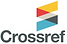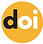top of page## ISSN 2622-9374Published: 22 June 2023

# On Operators Preserves in Normed Inner Product Spaces

Bamyan University, Afghanistan10.5281/zenodo.8063579

Pages: 92-98

Keywords: Convex, Operators, Orthogonality, Norm Space, Linear Operators, Inner Product Space

Abstract

We consider that a ﬁnite dimensional real normed linear space X is an inner product space if for any linear operator T on X, T preserving its norm at e1,e2∈SX implies T attains its norm at span{e1,e2}∩SX . We prove by the convexity theorem.

References

1. Alonso, J., Martini, H., and Wu, S. (2012). On birkhoff orthogonality and isosceles orthogonality in normed linear spaces. Aequationes mathematicae, 83(1-2):153–189.

2. Alsina, C., Sikorska, J., et al. (2010). Norm derivatives and characterizations of inner product spaces. World Scientific.

3. Bhatia, R. and Šemrl, P. (1999). Orthogonality of matrices and some distance problems. Linear algebra and its applications, 287(1-3):77–85.

4. Birkhoff, G. (1935). Orthogonality in linear metric spaces.

5. Blanco, A. and Turnšek, A. (2006). On maps that preserve orthogonality in normed spaces. Proceedings of the Royal Society of Edinburgh Section A: Mathematics, 136(4):709–716.

6. Carlsson, S. O. (1962). Orthogonality in normed linear spaces. Arkiv för Matematik, 4(4):297–318.9

7. Chmieliński, J. (2005). Linear mappings approximately preserving orthogonality. Journal of mathematical analysis and applications, 304(1):158–169.

8. Chmieliński, J. and Wójcik, P. (2010). Isosceles-orthogonality preserving property and its stability. Nonlinear Analysis:Theory, Methods & Applications, 72(3-4):1445–1453.

9. CONWAY, J. (1985). A course in functional analysis. Coleção graduate texts in mathematics, 96. Springer-Verlag: New York, 75:76.

10. Li, C.-K. and Schneider, H. (2002). Orthogonality of matrices. Linear algebra and its applications, 347(1-3):115–122.

11. Lumer, G. (1961). Semi-inner-product spaces. Transactions of the American Mathematical Society, 100(1):29–43.

12. Mojškerc, B. and Turnšek, A. (2010). Mappings approximately preserving orthogonality in normed spaces. Nonlinear Analysis: Theory, Methods & Applications, 73(12):3821–3831.

13. NSKI, J. C. and AZYCH, P. (2005). On an ε-birkhoff orthogonality. J. Inequal. Pure Appl. Math, 6(3).

14. Paul, K., Sain, D., and Ghosh, P. (2016a). Birkhoff–james orthogonality and smoothness of bounded linear operators. Linear Algebra and its Applications, 506:551–563.

15. Paul, K., Sain, D., and Ghosh, P. (2016b). Birkhoff–jamesorthogonality and smoothness of bounded linear operators. Linear Algebra and its Applications, 506:551–563.

16. Sain, D. (2017). Birkhoff–james orthogonality of linear operators on finite dimensional banach spaces. Journal of Mathematical Analysis and Applications, 447(2):860–866.

17. Turnšek, A. (2005). On operators preserving james’ orthogonality. Linear algebra and its applications, 407:189–195.

18. Watson, G. A. (1992). Characterization of the subdifferential of some matrix norms. Linear algebra and its applications, 170(0):33–45.

19. Wójcik, P. (2012). Linear mappings preserving ρ-orthogonality. Journal of Mathematical Analysis and Applications, 386(1):171–176.

20. Wójcik, P. (2019). Mappings preserving b-orthogonality. Indagationes Mathematicae, 30(1):197–200.

bottom of page